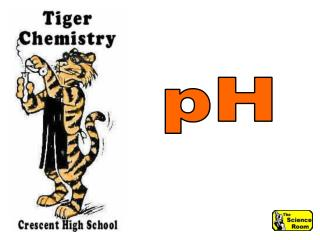DownloadDownload PresentationPotential Hydrogen

# Potential Hydrogen

Télécharger la présentation## Potential Hydrogen

- - - - - - - - - - - - - - - - - - - - - - - - - - - E N D - - - - - - - - - - - - - - - - - - - - - - - - - - -
##### Presentation Transcript

1. pH

2. pH Potential Hydrogen

3. pH A measure of hydronium ion concentration

4. pH A measure of [H3O+]

5. pH of some solutions HCl - 0 Stomach acid - 2 Cola drinks - 3 Tomatoes - 4 Coffee - 5 Milk - 6.5

6. pH of some solutions HCl - 0 Stomach acid - 2 Cola drinks - 3 Tomatoes - 4 Coffee - 5 Milk - 6.5 Pure water - 7 Blood - 7.5 Sea water - 8 Detergent - 10 Household cleaners - 11 Oven cleaners - 14

7. pH Scale - 17 min

8. Measuring pH Meters Indicators strips liquids

9. Measuring pH Most professional laboratories use a digital pH meter to read the pH of solutions directly.

10. Measuring pH These instruments provide a very quick and precise reading.

11. Measuring pH Bench-type pH meters have an electrode on an adjustable arm and are capable of large-scale use, with results to five significant figures.

12. Measuring pH Portable pH meters are battery-powered for use in the field.

13. Measuring pH They are obviously less expensive, but still provide readings with two or three significant figures.

14. Measuring pH These are common in high school chem labs.

15. Measuring pH Before pH meters, chemical indicators were used to determine pH.

16. Measuring pH Indicators are weak organic acids and bases whose colors differ from the colors of their conjugate acids or bases.

17. Measuring pH Indicators have two major weaknesses:

18. Measuring pH • They only provide a "ballpark" determination of a solution's pH.

19. Measuring pH 2. They only work well with solutions that begin colorless.

20. pH Indicators Litmus paperis the simplest test strip, indicating only if a solution is an acid or base.

21. pH Indicators Red litmus turns blue in a base. pH > 8.5

22. pH Indicators Blue litmus turns red in an acid. pH < 5.5

23. pH Indicators DO NOT dip test papers into solutions.

24. pH Indicators This MAY contaminate your sample.

25. pH Indicators Touch a clean stirring rod to the sample, then touch the rod to the test paper.

26. Measuring pH Hydrion paper (pH paper) is similar to litmus paper, but provides a range of values.

27. Measuring pH The color changes are shown on the container.

28. Measuring pH pH paper is available in several ranges, for use in different types of solutions.

29. pH Indicators Phenolphthalein solution Colorless in acid Faintly pink at neutral Dark pink in base

30. pH Indicators Universal Indicator solution A solution that changes to these colors

31. Ionization of Water 2 H2O H3O+(aq) + OH-(aq)

32. The Ion Product Constant of Water Kw = [H3O+] [OH-] = 1 X 10-14

33. Calculating pH pH = - log [ H3O+ ] [H3O+] is expressed in powers of 10 from 10-14 to 100

34. Sample Problem #1 What is the pH of a solution with a [H3O+] of 1 x 10-4M?

35. Problem Solution p H = - log [H3O+] p H = - log (1 x 10-4)

36. Problem Solution p H = - log [H3O+] p H = - log (1 x 10-4) Do the following on your calculator: Enter 1x10-4 Press "LOG" PRESS "+/-" P H = ?

37. Problem Solution p H = - log [H3O+] p H = - log (1 x 10-4) Do the following on your calculator: Enter 1x10-4 Press "LOG" PRESS "+/-" P H = 4

38. Sample Problem #2 0.01 moles of HCl is added to water to make 1 dm3 of solution. Assuming the HCl is completely ionized, what is the pH of the solution?

39. Problem Solution p H = - log [H3O+] What is the [H3O+]?

40. Problem Solution p H = - log [H3O+] p H = - log (1 x 10-2)

41. Problem Solution p H = - log [H3O+] p H = - log (1 x 10-2) Do the following on your calculator: Enter 1x10-2 Press "LOG" PRESS "+/-" P H = ?

42. Problem Solution p H = - log [H3O+] p H = - log (1 x 10-2) Do the following on your calculator: Enter 1x10-2 Press "LOG" PRESS "+/-" P H = 2

43. Have you noticed anythingabout these two problems?

44. 1 X 10-7 If this # is 1

45. 1 X 10-7 If this # is 1 The pH is this as a positive #

46. Find the pH of a solution whose [H3O+] is 9.5 x 10-8 M. pH = - log [H3O+] Sample Problem #3

47. Find the pH of a solution whose [H3O+] is 9.5 x 10-8 M. pH = - log [H3O+] pH = - log (9.5 x 10-8) Sample Problem #3

48. Find the pH of a solution whose [H3O+] is 9.5 x 10-8 M. pH = - log [H3O+] pH = - log (9.5 x 10-8) pH = - (log 9.5 + log 10-8) Sample Problem #3